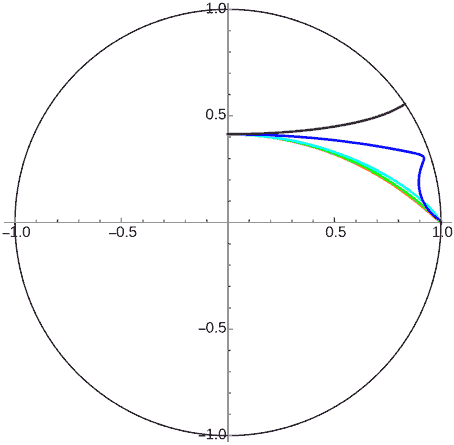# 神奇的双曲世界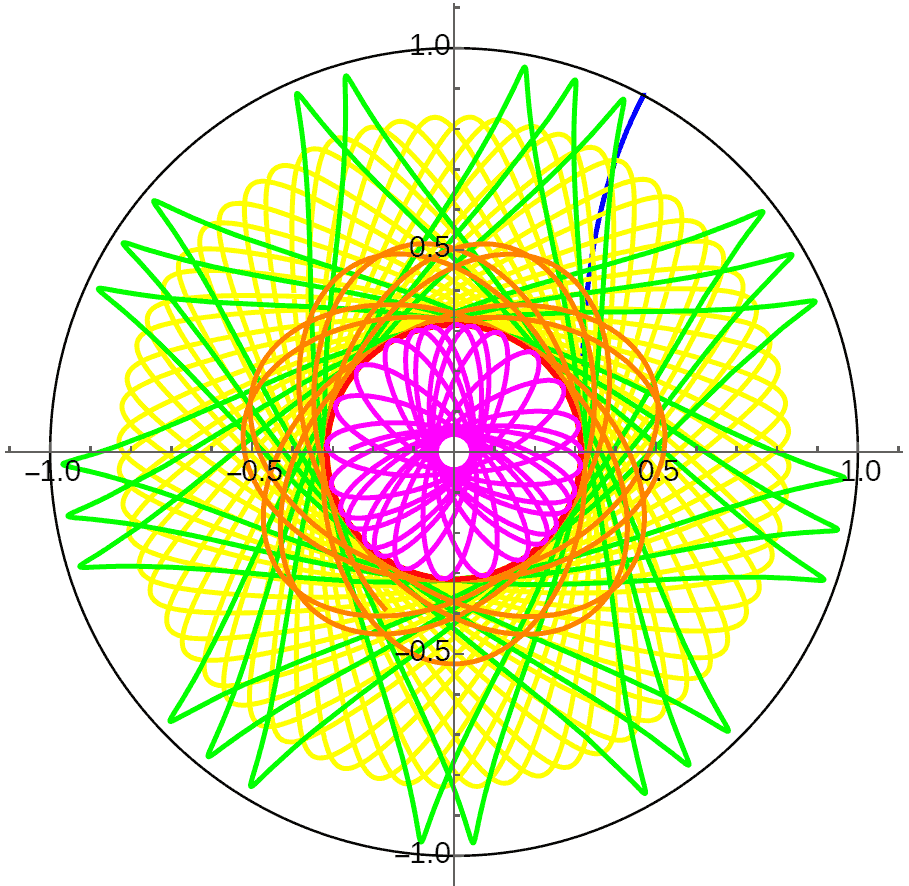## 直线、圆、极限圆与等距曲线

### 直线### 圆周1. 球面上的一般的圆周由不过球心的平面截球面得到，类似地，我们可用不过原点的平面去截双曲面，但与球面情况不同的是，截线有可能封闭，也有可能不封闭。如果截线是封闭图形，那么它就是圆。为什么呢？很明显我们用垂直于双曲面对称轴的平面切曲面，会得到一系列圆周，容易验证它们在闵氏几何度量下也是圆周，对于任意的平面，我们总能通过双曲旋转将它转到垂直于双曲面对称轴，所以就验证了这些封闭图形都是圆。
2. 双曲几何中的圆周通过庞加莱圆盘投影后依然是圆周，这类似球极投影的保圆性，但它们投影后圆的圆心与圆心实际位置往往不再重合。

### 极限圆1. 极限圆在庞加莱圆盘投影中与圆盘边缘相切。注意不要以为从极限圆上的相反方向走最终会相互靠近，这只是投影压缩边缘带来的错觉，事实上只会越走越远。
2. 极限圆跟圆一样高度对称的图形，且所有极限圆的曲率都相同，从这个意义上可以说它们都是一样大的。（下图明明是史密斯圆图）3. 三维闵氏空间中，极限圆是由平行于双曲面任意渐近线的平面截得的抛物线。## 双曲宇宙构想

1. 双曲世界中的导航很难，很容易迷失方向。（在Hyperrogue游戏中应该早已体会）
2. 一颗小小半径的星球的表面积、体积异常的大，几乎是个天文数字。

### 双曲世界中的刚体运动

#### 平移#### 旋转### 场与高斯定理### 球面宇宙悖论## 球面几何相关公式推导

### 球面圆周长公式推导

1. 球面圆周长随半径先增大后减小，当半径为$\pi$时圆的周长变成了0，此时圆周已经在圆心的对面缩成了一个点。
2. 当半径很小时，$\sin(r)\approx r$，球面圆周长公式与平面圆周长公式相同。

### 球面圆面积公式推导

$$S(r)=\int_0^r2\pi u \mathrm{d} u=\pi r^2$$

$$S(r)=\int_0^r2\pi \sin(u) \mathrm{d} u=2\pi(1-\cos(r))$$

## 双曲面模型

### 双曲圆周长公式推导

$$x^2+y^2-z^2=-1$$

### 双曲圆面积公式推导

$$S(r)=\int_0^r2\pi \sinh(u) \mathrm{d} u=2\pi(\cosh(r)-1)$$

### 双曲等距曲线距离公式推导## 对称星球的引力分布

### 圆形星球的外部引力分布### 圆形星球的内部引力分布

$${(\cosh(r)-1)\sinh(R)\over(\cosh(R)-1)\sinh(r)}$$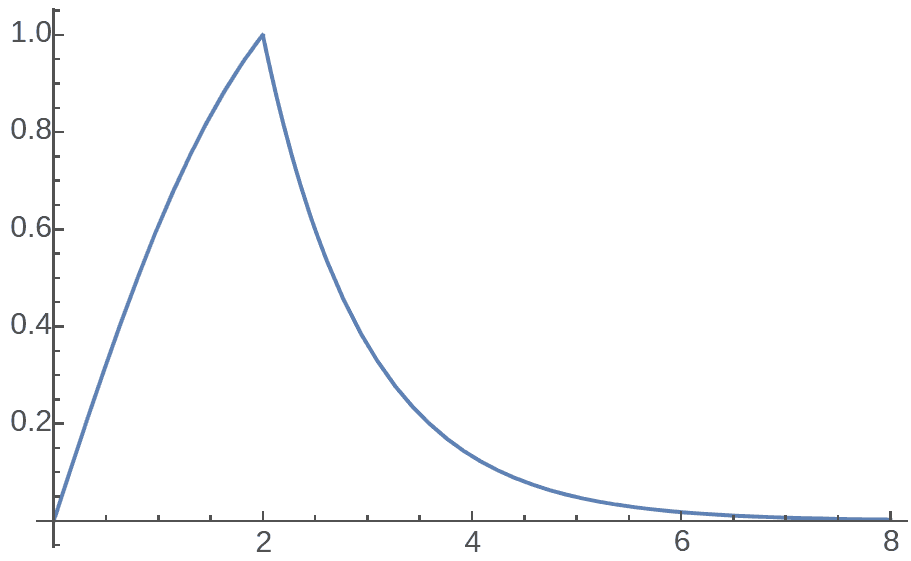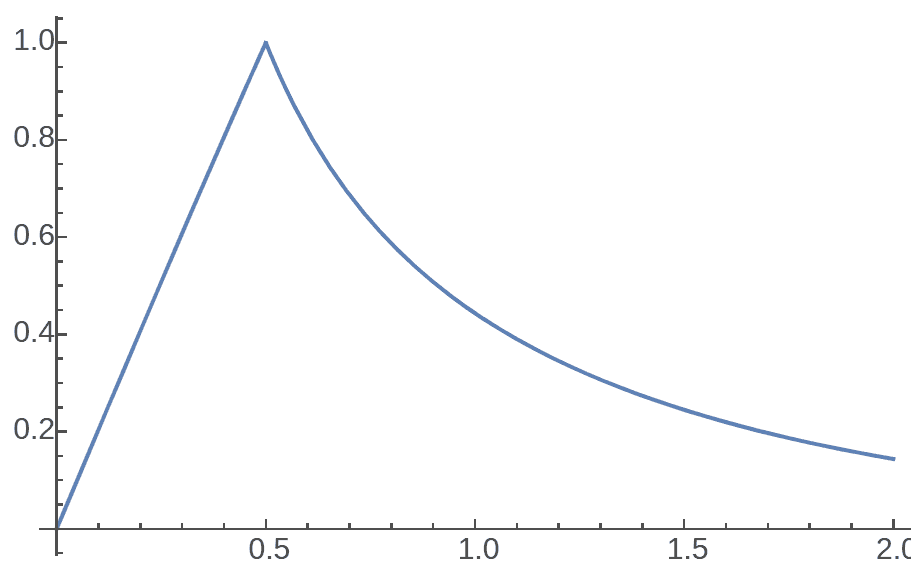### 极限圆星球的引力分布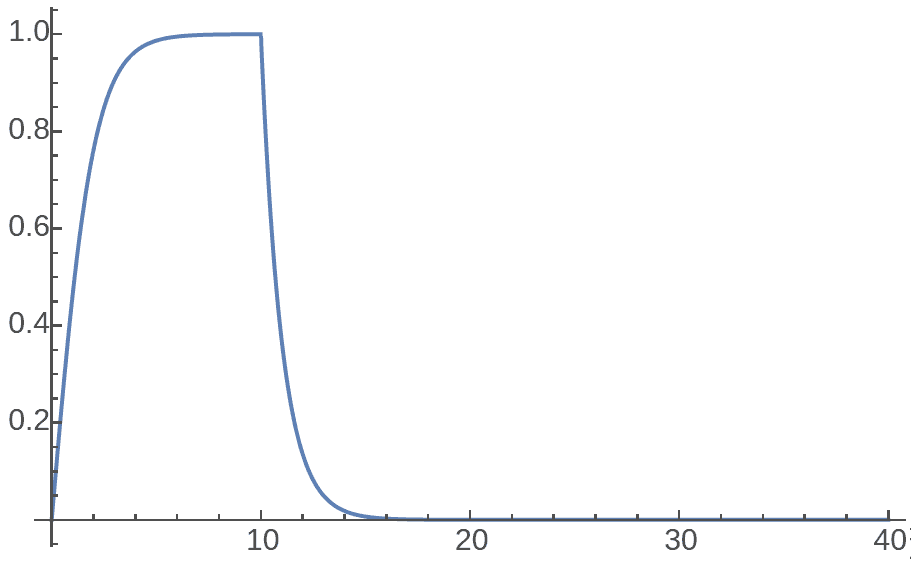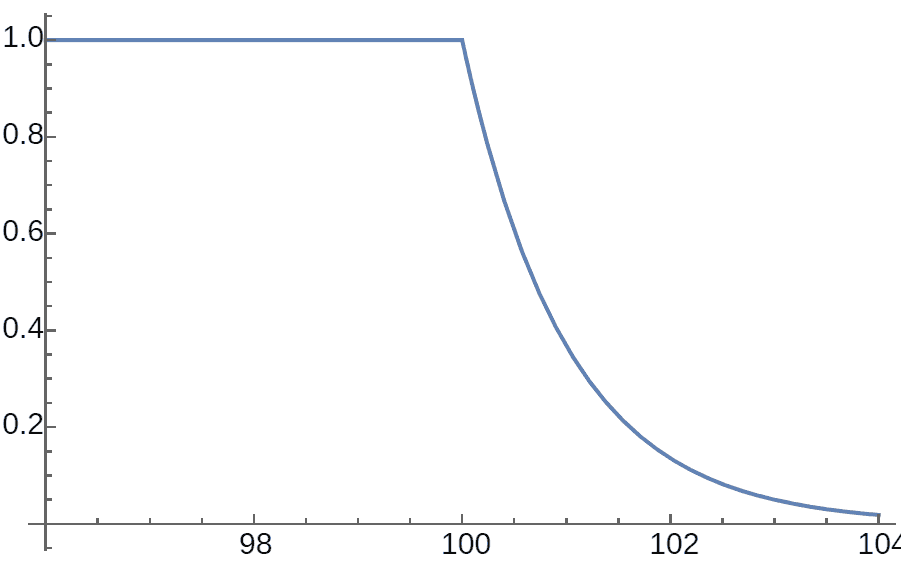### 半平面星球的内部引力分布## 三维双曲宇宙

• 半径为$r$的球的表面积：$4\pi \sinh^2(r)$
• 半径为$r$的球的体积：$\pi(\sinh(2r)-2r)$
• 相距为$d$的等距曲面与测地面的面积比：$\cosh^2(d)$

• 半径为$R$的星球距球心r处的场强：
$$r<R:k{(\sinh(2r)-2r)\sinh^2(R)\over(\sinh(2R)-2R)\sinh^2(r)}$$
$$r>R:k{\sinh^2(R)\over\sinh^2(r)}$$
• 距极限球表面距离为r处的场强：
内部：$k$

外部：$k\exp(-2r)$### 极限球星球的几何

1. 这颗极限球星球上的测地线是什么呢？想象球面上的赤道和小圆，显然赤道是“直”的，没有曲率，而小圆则有。极限球星球也相当于一种双曲宇宙上的“小球”，它表面是有曲率且是常曲率的。极限球是普通球的极限，那么它上面最直的线（即测地线）自然就是大圆，又由于极限球的球心在无穷远处，所以这些大圆都是通往无穷的极限圆。
2. 仿照球面三角形、球面圆，我们也可以定义一种在极限球表面上的极限球面圆，它的周长与半径之间的关系是什么呢？计算发现周长公式居然就是$2\pi r$，与平面情况完全相同，这是怎么回事呢？首先极限球面圆的半径$r$是极限圆上的一段弧长，这个圆在真正的双曲空间中的半径应该是极限球内部的一条弦的一半，即图中$r’$。我们知道双曲空间中周长与半径成指数关系，即$r’$较短的时候圆的周长却可以很长，同理当弦较短的时候对应的极限圆弧也可以很长，这两个指数关系约掉后，在极限球面上反而恢复成了跟平直的二维平面相同的情况。其实极限球表面的度量完全与二维欧氏平面等价，这颗星球的世界地图可以完全绘制在平面上且保证任何地方都没有扭曲！可惜这幅零曲率的世界地图反而却无法很好地放进星球所在的负曲率双曲宇宙中……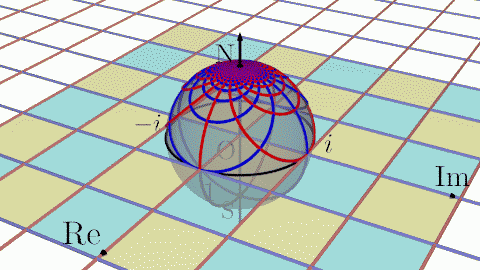### 天体运动的稳定性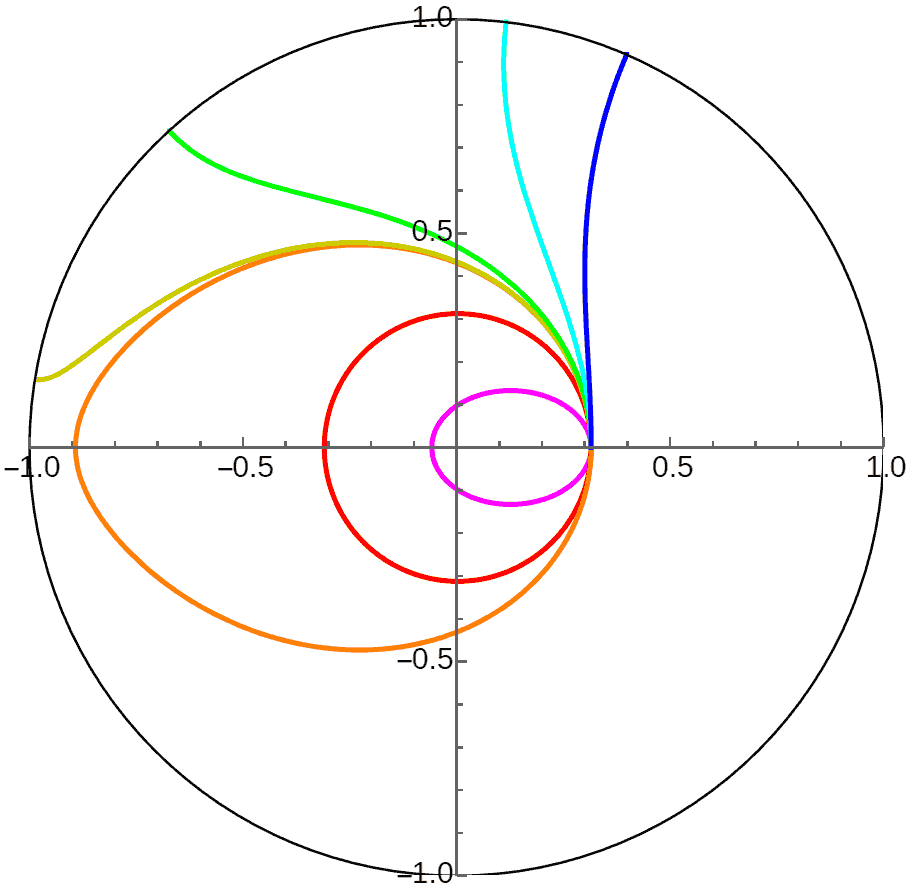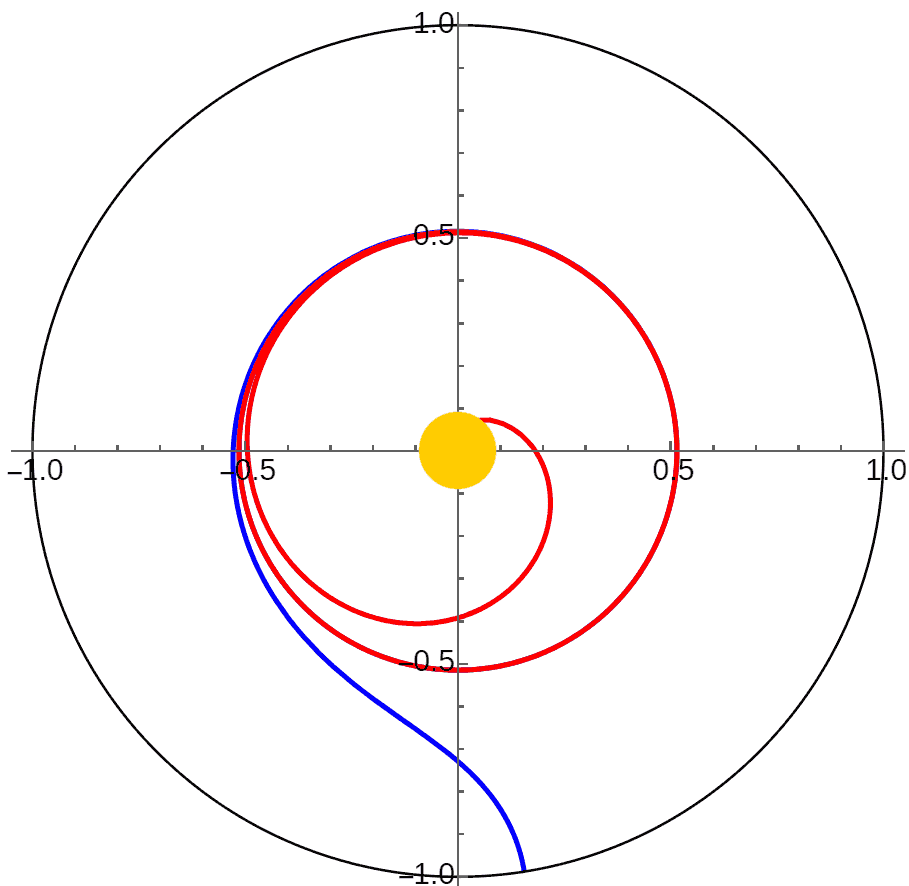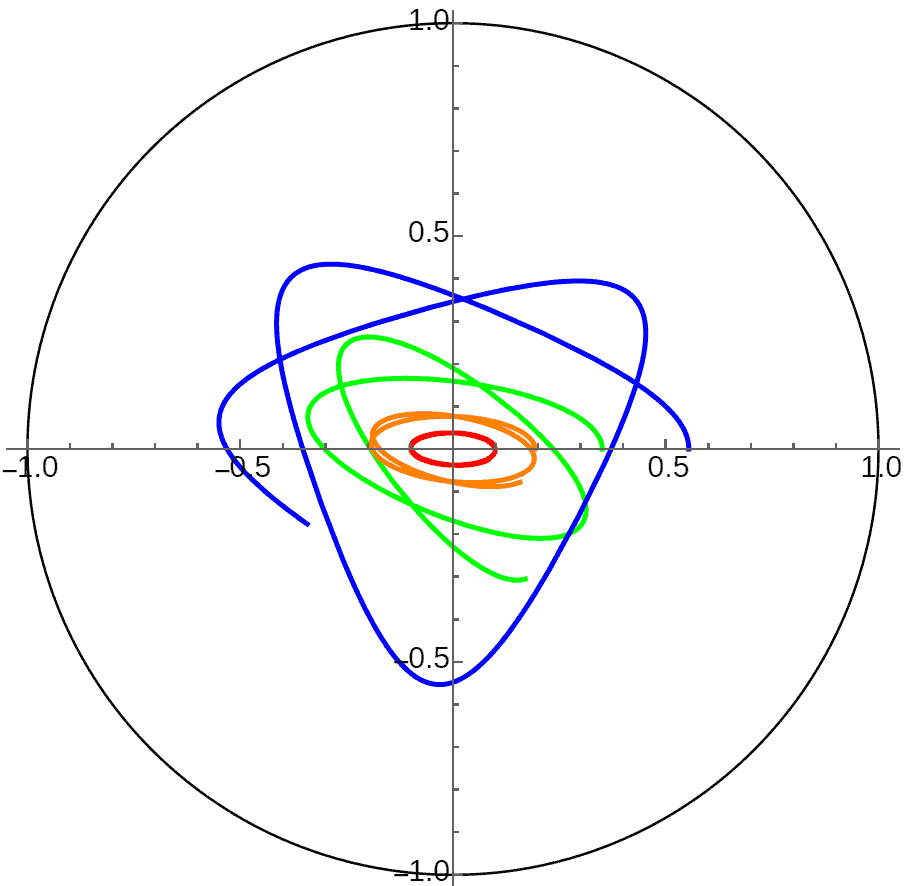### 中心天体位于无限远处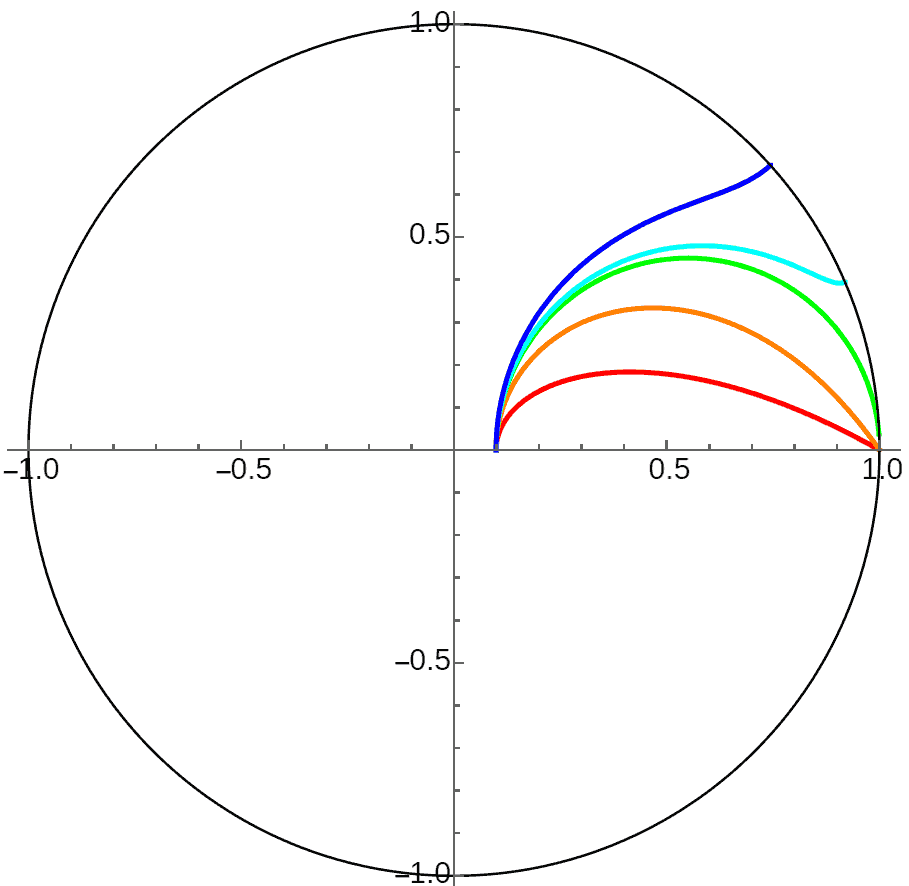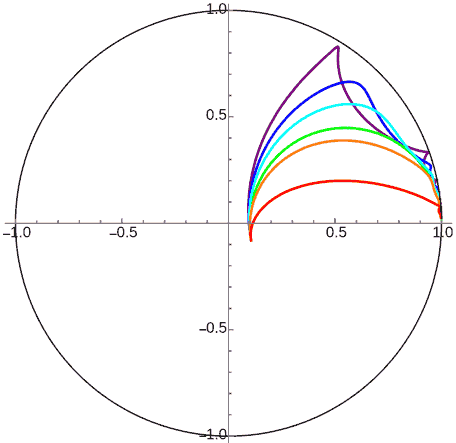#### 行星位于极限球星球内部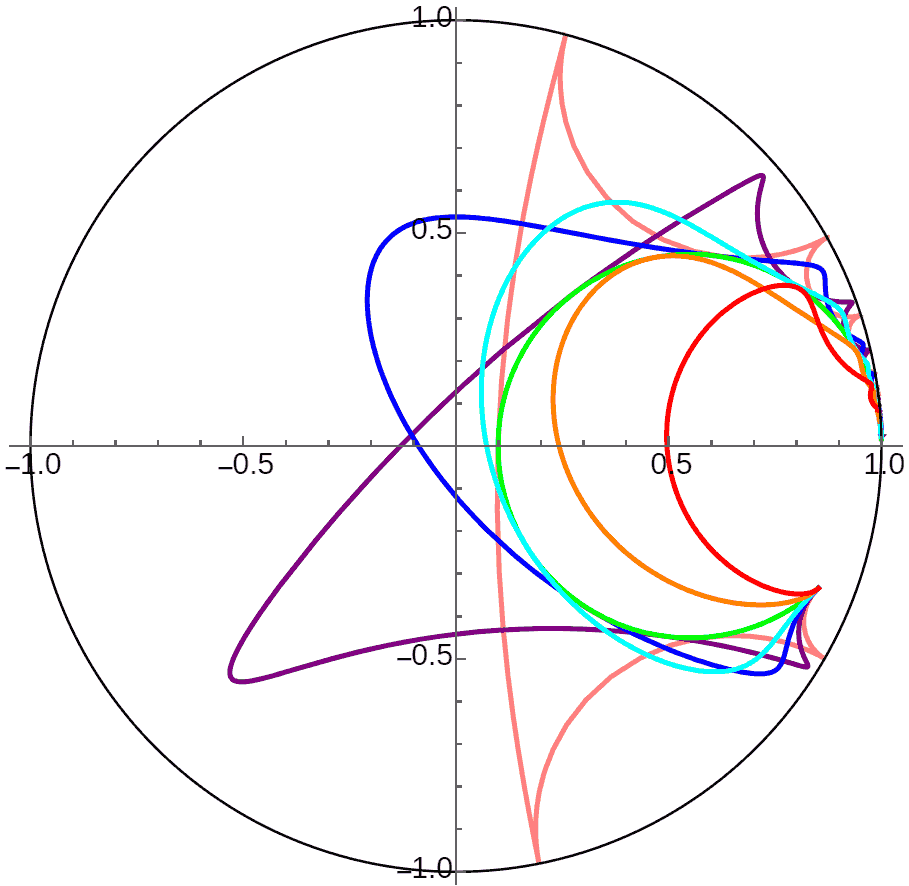### 行星环绕空壳无限大平面星球